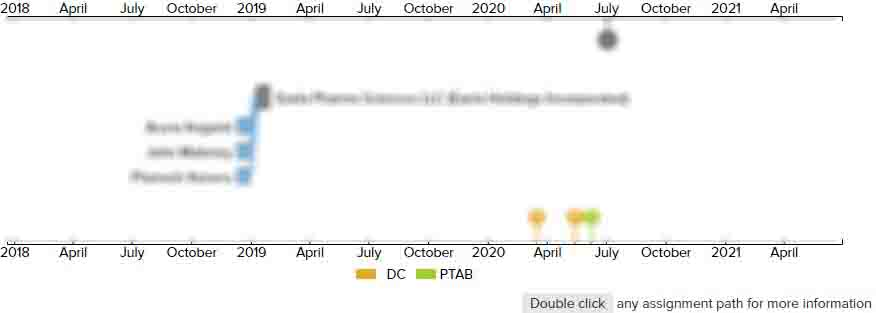# Method of PCR testing of pooled blood samples

• US 6,063,563 A
• Filed: 05/20/1998
• Issued: 05/16/2000
• Est. Priority Date: 04/10/1995
• Status: Expired due to Term
##### First Claim
Patent Images

1. A method for uniquely identifying viral positive biological fluid donations in the fewest number of high-sensitivity test cycles, the method comprising:

• providing a multiplicity of biological fluid donations;

defining an n-dimensional matrix, where n is an integer, the matrix further comprising a multiplicity of elements, each element defined by an intersection of the n-dimensions of the matrix, each individual element identified by a respective matrix notation, the matrix notation comprising at least an index for each dimension of the array;

taking a sample from each of the multiplicity of biological fluid donations;

mapping each sample to a respective particular one of each element of the matrix, each individual sample identified by its corresponding element'"'"'s respective matrix notation;

taking aliquots from each sample, the number of aliquots taken from each sample defined by the number of dimensions characterizing the matrix;

forming subpools from the aliquots of each sample, each subpool containing an aliquot from all samples identified by a matrix notation in which one dimensional index is fixed, each respective subpool identified by said fixed dimensional index;

providing the subpools to a high-sensitivity testing facility, wherein all of the subpools are tested for viral indication in a single high-sensitivity test cycle;

determining the respective fixed dimensional indices of subpools which return a positive viral indication; and

combining said fixed dimensional indices into a matrix notation, thereby unambiguously identifying a unique matrix element defined by the matrix notation, thus unambiguously identifying a uniquely viral positive sample.

• ##### 8 Assignments
Timeline View
Assignment View×
×